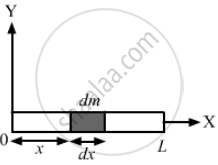Department of Pre-University Education, KarnatakaPUC Karnataka Science Class 11

# A Rod of Length L is Placed Along the X-axis Between X = 0 and X = L. the Linear Density (Mass/Length) ρ of the Rod Varies with the Distance X from the Origin as ρ = a + Bx. - Physics

Sum

A rod of length L is placed along the X-axis between x = 0 and x = L. The linear density (mass/length) ρ of the rod varies with the distance x from the origin as ρ = a + bx. (a) Find the SI units of a and b. (b) Find the mass of the rod in terms of a, b and L.

#### Solution

ρ = mass/length = a + bx

So, the SI unit of ρ is kg/m.

(a)
SI unit of a = kg/m
SI unit of b = kg/m2
(From the principle of homogeneity of dimensions)

(b) Let us consider a small element of length dx at a distance x from the origin as shown in the figure given below:dm = mass of the element
= ρdx
= (a + dx) dx
∴ Mass of the rod = ∫ dm

$= \int_0^L \left( a + bx \right) dx$

$= \left[ ax + \frac{b x^2}{2} \right]_0^L$

$= aL + \frac{b L^2}{2}$

Concept: Physics Related to Technology and Society
Is there an error in this question or solution?

#### APPEARS IN

HC Verma Class 11, Class 12 Concepts of Physics Vol. 1
Chapter 2 Physics and Mathematics
Exercise | Q 27 | Page 29

Share